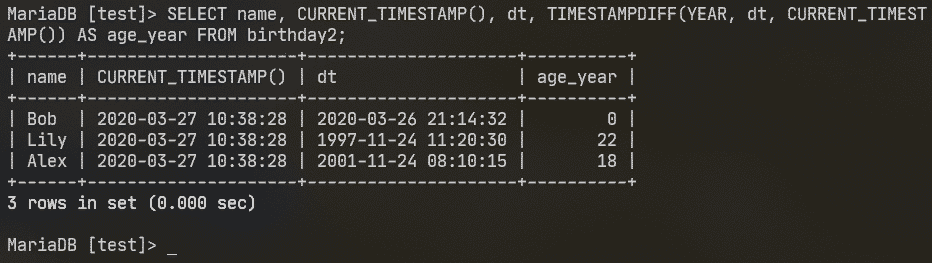# Working with MySQL-MariaDB Date and Time

In this article, I am going to show you how to work with MySQL/MariaDB DATE, TIME and DATETIME data types. So, let’s get started.

### Prerequisites:

You must have MySQL or MariaDB installed on your Linux OS (i.e. CentOS/RHEL, Ubuntu/Debian). If you need any assistance on installing MySQL/MariaDB, there are many articles on LinuxHint.com which you can check.

### Creating Dummy Tables and Rows:

I will use a birthday table for demonstrating how to work with DATE and TIME data types in this article. The birthday table has a name field of type VARCHAR, a date field of type DATE and a time field of type TIME.

You can create the birthday table as follows.

> CREATE TABLE birthday (
name VARCHAR(20) NOT NULL,
date DATE,
time TIME
);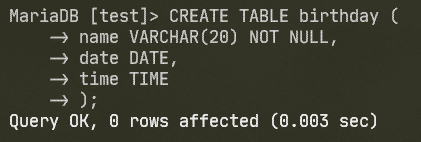If you want MySQL/MariaDB to automatically add the current date or current time while inserting new rows into the birthday table, you can create the birthday table as follows.

Here, DEFAULT CURRENT_DATE automatically adds the current date to the date colum if no data is provided for that column while insertion. The same way DEFAULT CURRENT_TIME automatically adds the current time to the time column.

### Working with Date:

You can print the current date with the CURRENT_DATE() function as follows: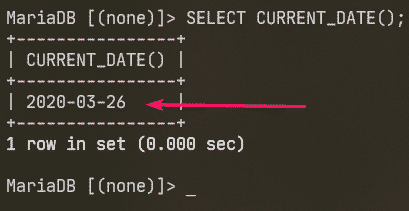If ‘Bob’ was born today, you can add ‘Bob’ into the birthday table as follows:

> INSERT INTO birthday(name, date) VALUES('Bob', CURRENT_DATE());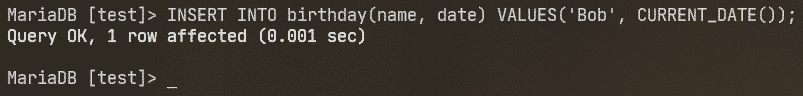You can also add specific birth dates as follows:

> INSERT INTO birthday(name, date) VALUES('Lily', '1997-11-24');
> INSERT INTO birthday(name, date) VALUES('Alex', '2001-11-24');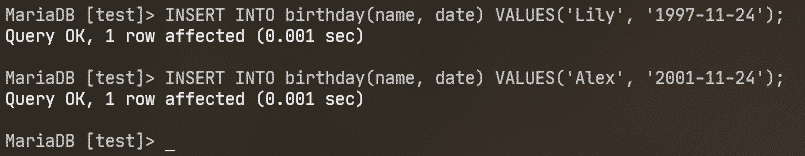The current state of the birthday table is as follows.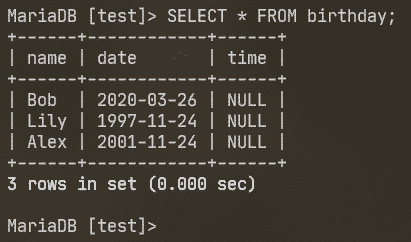You can extract only the year part of the date using the YEAR() function, the month part using the MONTH() function, the day part using the DAY() function as follows:

> SELECT name, YEAR(date), MONTH(date), DAY(date) FROM birthday;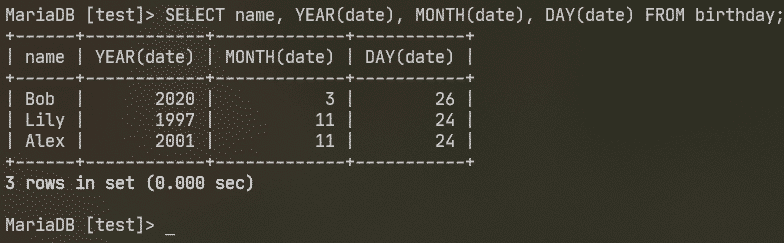You can find month name of a date using MONTHNAME() function.

> SELECT name, date, MONTHNAME(date) FROM birthday;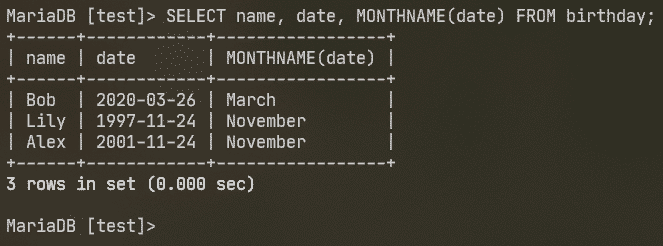1 year is equal to 52 weeks. You can find the week of the year using the WEEKOFYEAR() function as follows:

> SELECT name, date, WEEKOFYEAR(date) FROM birthday;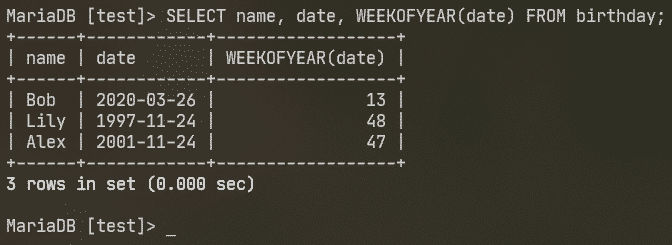The same way, you can get the day of the year using the DAYOFYEAR() function. 1 year is equal to 365 days. 366 days in a leap year.

> SELECT name, date, DAYOFYEAR(date) FROM birthday;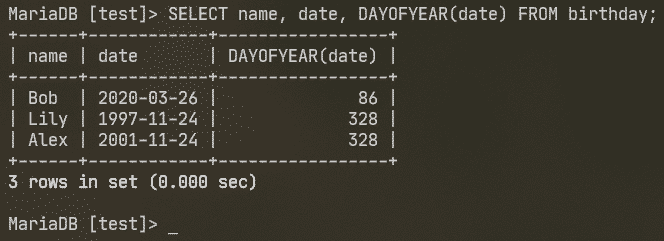You can find the week day from a date using the WEEKDAY() function.

> SELECT name, date, WEEKDAY(date) FROM birthday;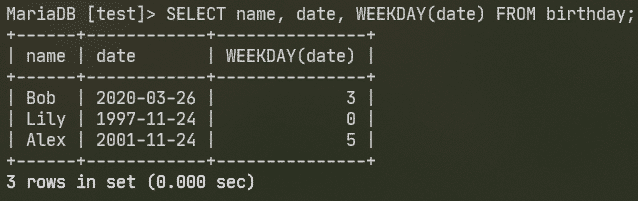Here, 0 is Monday, 1 is Tuesday, 2 is Wednesday, 3 is Thursday, 4 is Friday, 5 is Saturday and 6 is Sunday.

You can also find the week day name using the DAYNAME() function.

> SELECT name, date, DAYNAME(date) FROM birthday;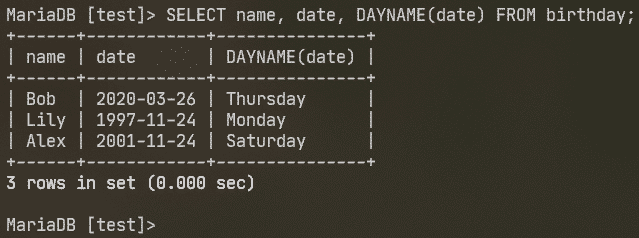### Working with Time:

You can find the current system time using the CURRENT_TIME() function as follows.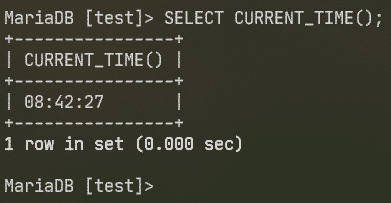The time column of our birthday table is NULL at this point.

> SELECT * FROM birthday;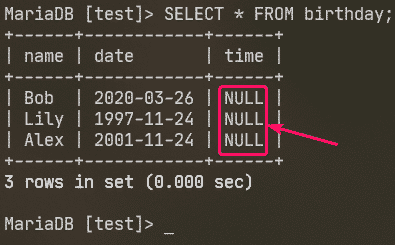Let’s add some dummy time values to the time column.

> UPDATE birthday SET time='21:14:32' WHERE name='Bob';
> UPDATE birthday SET time='11:20:30' WHERE name='Lily';
> UPDATE birthday SET time='8:10:15' WHERE name='Alex';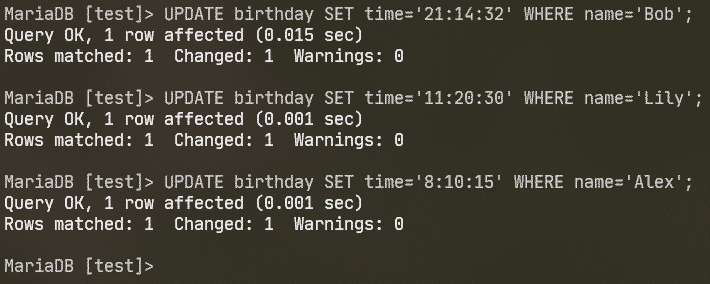Now, the birthday table should look something like this.

> SELECT * FROM birthday;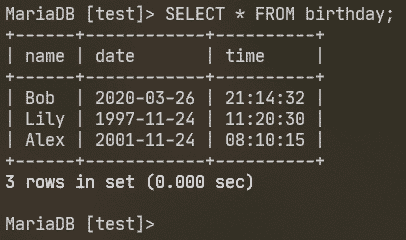You can find the hour of the time using the HOUR() function, the minute using the MINUTE() function, and the second using the SECOND() function as follows:

> SELECT name, HOUR(time), MINUTE(time), SECOND(time) FROM birthday;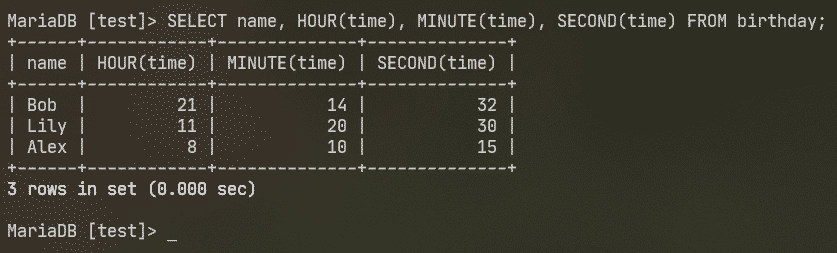### Working with Date & Time:

Earlier, I’ve stored the date and time in different fields of the birthday table. That’s impractical. If you need to store the date and time information, you should use the DATETIME data type.

You can create a new birthday table birthday2 that uses the DATETIME data type as follows:

> CREATE TABLE birthday2 (
name VARCHAR(20) NOT NULL,
dt DATETIME
);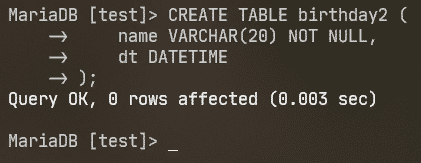Now, import data from the birthday table to birthday2 table as follows:

> INSERT INTO birthday2 SELECT name,
CONCAT(date, ' ', time) AS dt FROM birthday;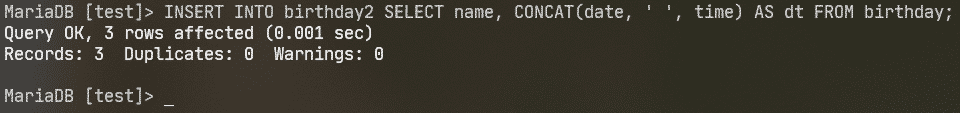This is how the birthday2 table should look like at this point.

> SELECT * FROM birthday2;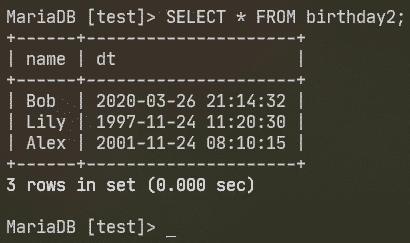You can convert the datetime to seconds (TIMESTAMP) using the TO_SECONDS() function as follows:

> SELECT name, TO_SECONDS(dt) FROM birthday2;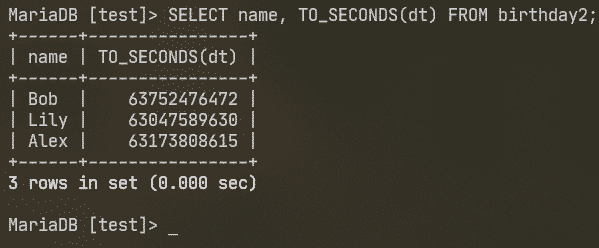All the functions I have used in the Working with Date and Working with Time sections of this article will also work on DATETIME fields.

The DATE_ADD() function is used to add to the date and DATE_SUB() function is used to subtract from the date. The fomat of DATE_ADD() and DATE_SUB() are the same.

The format of the DATE_ADD() function:

The format of the DATE_SUB() function:

DATE_SUB(dt, INTERVAL expr unit)

Here, INTERVAL is a keyword.

dt is the DATE, TIME or DATETIME to which you want to add to or subtract from.

unit can be YEAR, MONTH, DAY, WEEK, HOUR, MINUTE, SECOND.

expr is a numeric quanity of the defined unit.

For example, you can add an year to the date using the DATE_ADD() function as follows:

> SELECT name, dt, DATE_ADD(dt, INTERVAL 1 YEAR) FROM birthday2;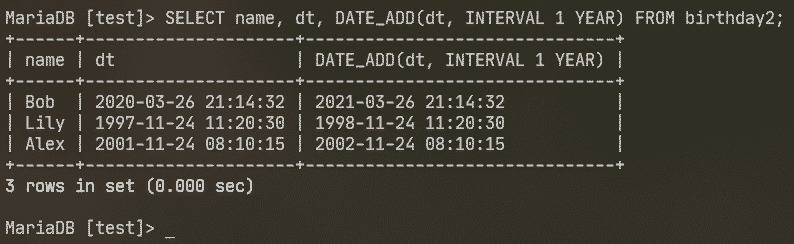The same way, you can subtract a month using the DATE_SUB() function as follows:

> SELECT name, dt, DATE_SUB(dt, INTERVAL 1 MONTH) FROM birthday2;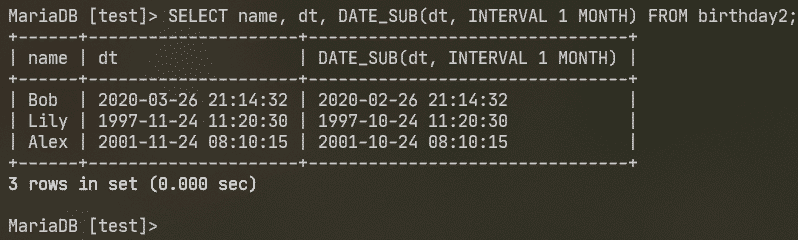### Finding the Difference Between 2 Dates:

You can find the difference between 2 dates using the TIMESTAMPDIFF() function.

The format of the TIMESTAMPDIFF() function is:

TIMESTAMPDIFF(unit, dt1, dt2)

Here, dt1 and dt2 can be of type DATE or DATETIME.

The TIMESTAMPDIFF() function returns (dt2dt1) in the defined unit.

The unit can be YEAR, MONTH, DAY, WEEK, HOUR, MINUTE, SECOND.

You can find the age (in seconds) of each person in the birthday table as follows:

> SELECT name, CURRENT_TIMESTAMP(), dt, TIMESTAMPDIFF(SECOND,
dt, CURRENT_TIMESTAMP()) AS age_seconds FROM birthday2;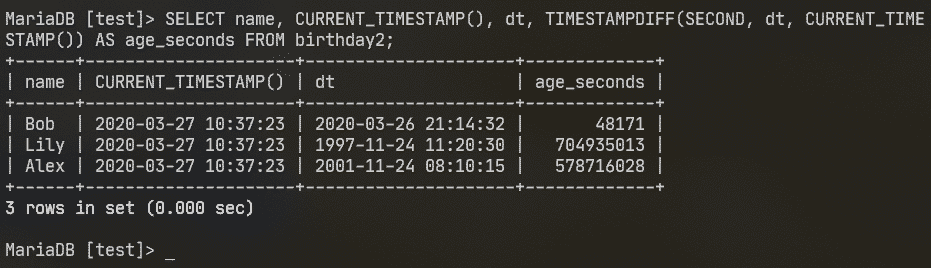The same way, you can find the age in days as follows:

> SELECT name, CURRENT_TIMESTAMP(), dt, TIMESTAMPDIFF(DAY,
dt, CURRENT_TIMESTAMP()) AS age_days FROM birthday2;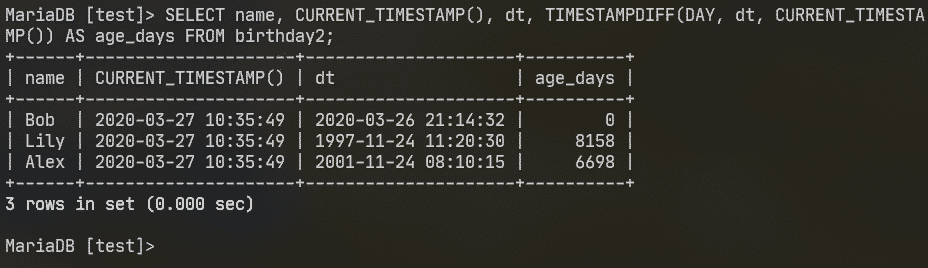You can also find the age in years as follows:

> SELECT name, CURRENT_TIMESTAMP(), dt, TIMESTAMPDIFF(YEAR, dt,
CURRENT_TIMESTAMP()) AS age_year FROM birthday2;